Select Page

February 2021

## Keep up those challenges every day!

Here are the answers to the February 2021 Brain Practice

### The Matchstick Challenge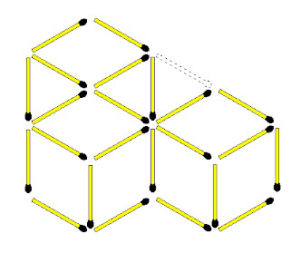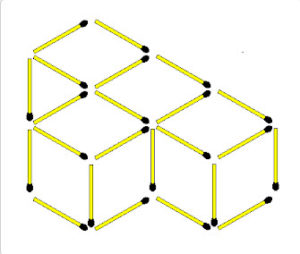### Rotating Figures Challenge. Find the Exception.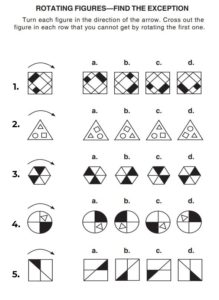### The Ball Challenge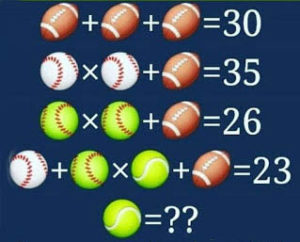Tennis Ball = 2

Explanation
Equation 1: 10 + 10 + 10 = 30   (Rugby Ball = 10)
Equation 2: (5 x 5) + 10 = 35  (White Baseball = 5)

Equation 3: (4 x 4) + 10 = 26  (Green Baseball = 4)

Let us assume that the value of ‘Tennis Ball’ be ‘T’;
Equation 4:
5 + (4 x T) + 10 = 23
=> 4T + 15 = 23
=> 4T = 23 – 15
=> 4T = 8
=> T = 8/4

=> T = 2

Therefore; the value of ‘Tennis Ball’ = 2.

### Verbal Challenge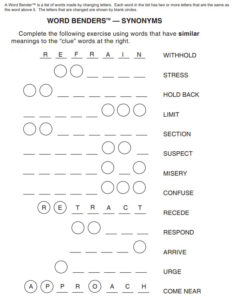1. STRAIN
2. RESTRAIN
3. RESTRICT
4. DISTRICT
5. DISTRUST
6. DISTRESS
7. DISTRACT
8. REACT
9. REACH
10. PREACH

## Can you solve these?

• A girl who was just learning to drive went down a one-way street in the wrong direction, but didn’t break the law. How is that possible?
She was walking!
• How can you share 5 apples with 5 people and still have one left in the basket?
One person carries the apple in the basket.
•
• There are several suggested answers to this one. Which is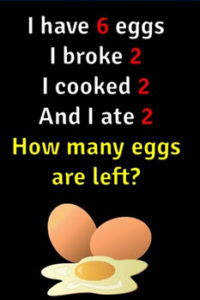correct, do you think?
– Grammar rules, the first sentence is in the present tense. So when it says he has 6 eggs, he has 6 eggs. The other sentences are in the past tense. (This is the officially correct answer ….)
However:
– The exact answer is 2 because if two eggs were broken from 6 eggs there will be 4 more and then she cooked and ate two extra eggs. 6-4=2 so there will be 2 more left

– There are four left… he broke two, cooked the same two, and ate the same two.

You decide for yourself!